## HashMap 概述

HashMap 是 Map 接口的实现，HashMap 允许空的 key-value 键值对，HashMap 被认为是 Hashtable 的增强版，HashMap 是一个非线程安全的容器，如果想构造线程安全的 Map 考虑使用 ConcurrentHashMap。HashMap 是无序的，因为 HashMap 无法保证内部存储的键值对的有序性。

HashMap 的底层数据结构是数组 + 链表的集合体，数组在 HashMap 中又被称为桶(bucket)。遍历 HashMap 需要的时间损耗为 HashMap 实例桶的数量 + (key – value 映射) 的数量。因此，如果遍历元素很重要的话，不要把初始容量设置的太高或者负载因子设置的太低。

HashMap 实例有两个很重要的因素，初始容量和负载因子，初始容量指的就是 hash 表桶的数量，负载因子是一种衡量哈希表填充程度的标准，当哈希表中存在足够数量的 entry，以至于超过了负载因子和当前容量，这个哈希表会进行 rehash 操作，内部的数据结构重新 rebuilt。

HashMap 会导致除了迭代器本身的 remove 外，外部 remove 方法都可能会导致 fail-fast 机制，因此尽量要用迭代器自己的 remove 方法。如果在迭代器创建的过程中修改了 map 的结构，就会抛出 ConcurrentModificationException 异常。

## HashMap 和 HashTable 的区别

### 相同点

HashMap 和 HashTable 都是基于哈希表实现的，其内部每个元素都是 key-value 键值对，HashMap 和 HashTable 都实现了 Map、Cloneable、Serializable 接口。

### 不同点

• 父类不同：HashMap 继承了 AbstractMap 类，而 HashTable 继承了 Dictionary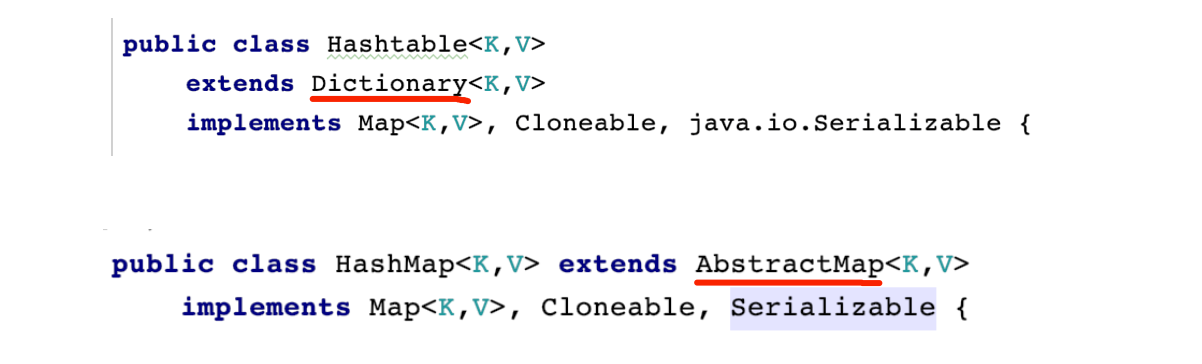• 空值不同：HashMap 允许空的 key 和 value 值，HashTable 不允许空的 key 和 value 值。HashMap 会把 Null key 当做普通的 key 对待。不允许 null key 重复。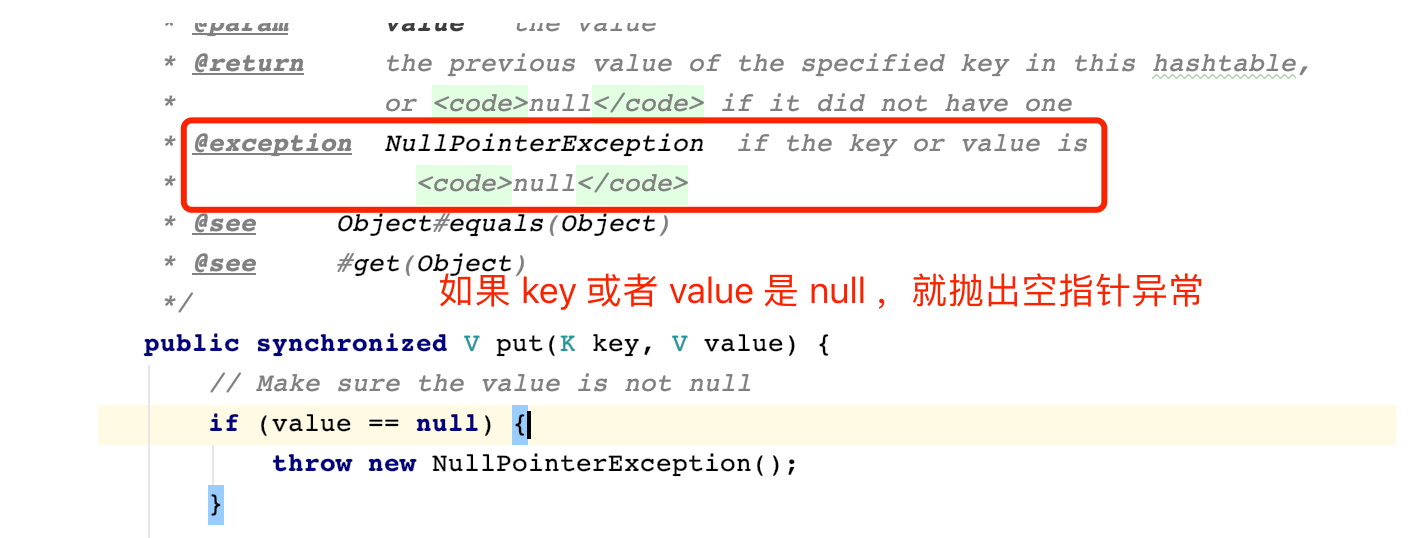• 线程安全性：HashMap 不是线程安全的，如果多个外部操作同时修改 HashMap 的数据结构比如 add 或者是 delete，必须进行同步操作，仅仅对 key 或者 value 的修改不是改变数据结构的操作。可以选择构造线程安全的 Map 比如 Collections.synchronizedMap 或者是 ConcurrentHashMap。而 HashTable 本身就是线程安全的容器。

• 性能方面：虽然 HashMap 和 HashTable 都是基于单链表的，但是 HashMap 进行 put 或者 get􏱤 操作，可以达到常数时间的性能；而 HashTable 的 put 和 get 操作都是加了 synchronized 锁的，所以效率很差。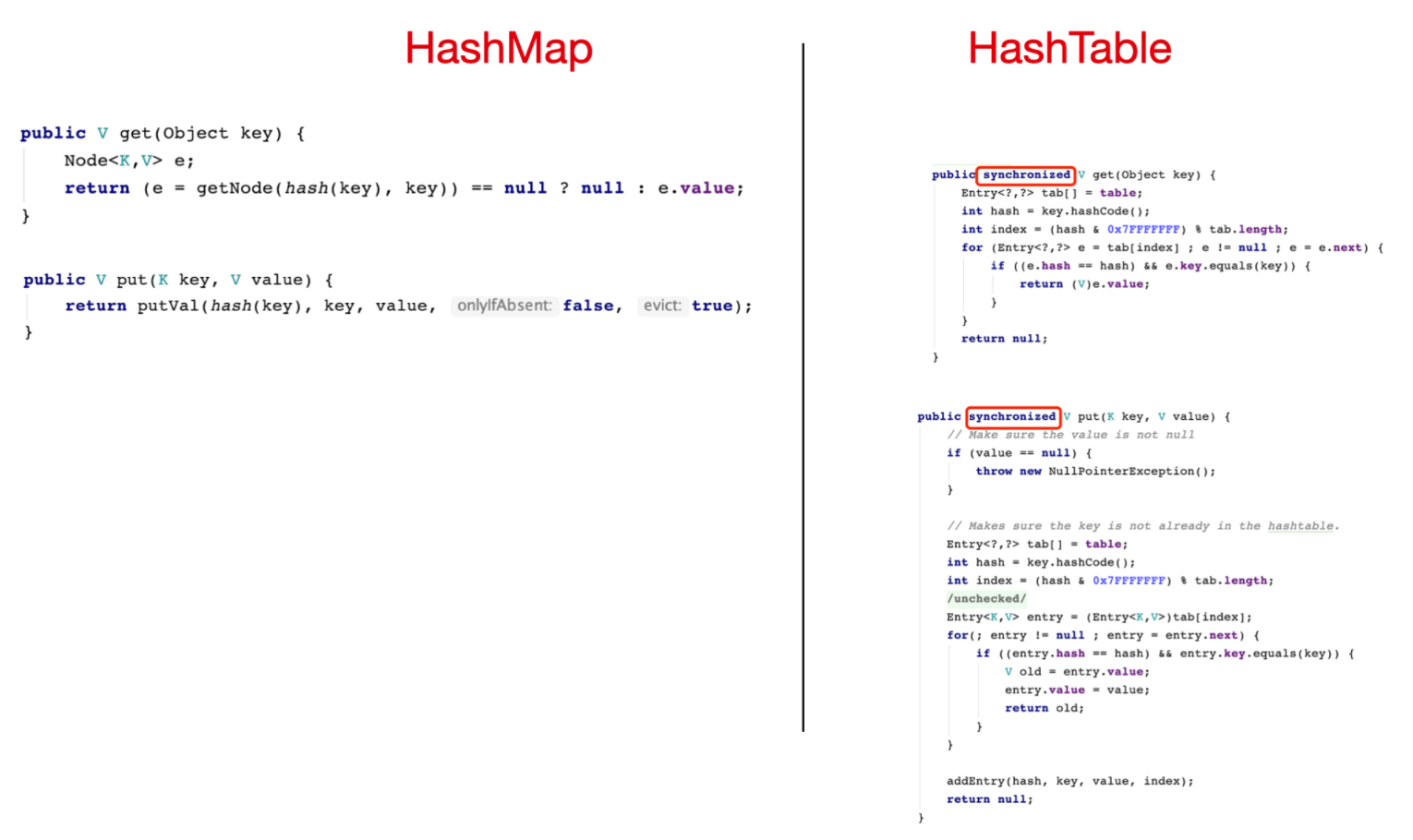• 初始容量不同：HashTable 的初始长度是11，之后每次扩充容量变为之前的 2n+1（n为上一次的长度）

而 HashMap 的初始长度为16，之后每次扩充变为原来的两倍。创建时，如果给定了容量初始值，那么HashTable 会直接使用你给定的大小，而 HashMap 会将其扩充为2的幂次方大小。

## HashMap 和 HashSet 的区别

HashSet 继承于 AbstractSet 接口，实现了 Set、Cloneable,、java.io.Serializable 接口。HashSet 不允许集合中出现重复的值。HashSet 底层其实就是 HashMap，所有对 HashSet 的操作其实就是对 HashMap 的操作。所以 HashSet 也不保证集合的顺序。

## HashMap 底层结构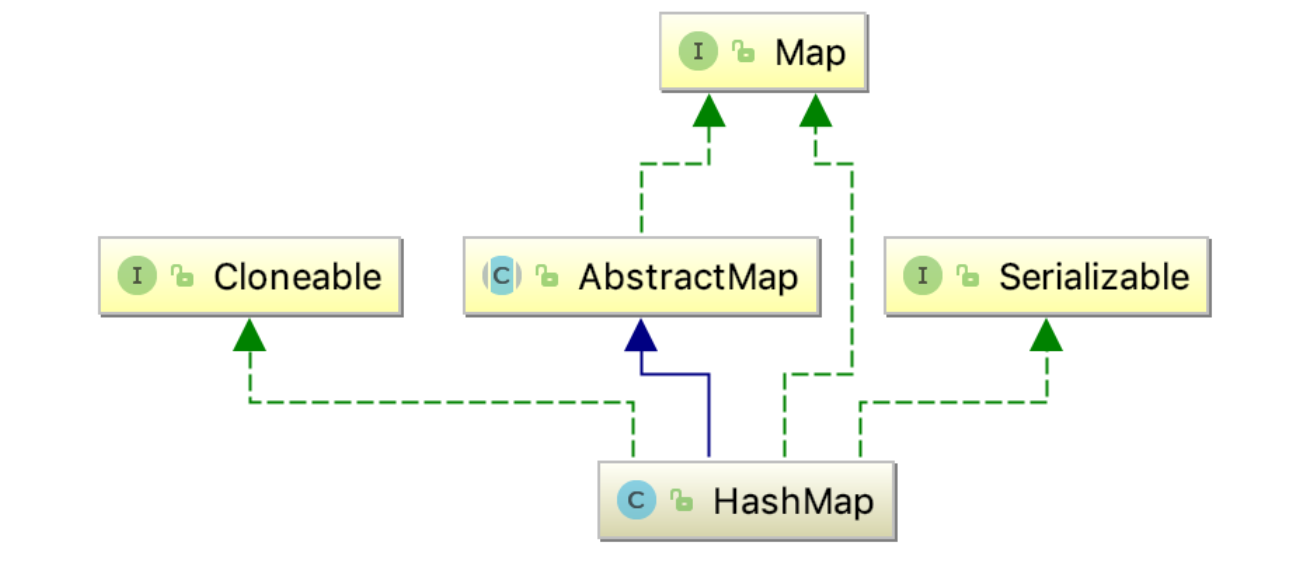### Map 接口

Map 接口定义了 key-value 键值对的标准。一个对象支持 key-value 存储。Map不能包含重复的 key，每个键最多映射一个值。这个接口代替了Dictionary 类，Dictionary是一个抽象类而不是接口。

Map 接口提供了三个集合的构造器，它允许将 map 的内容视为一组键，值集合或一组键值映射。map的顺序定义为map映射集合上的迭代器返回其元素的顺序。一些map实现，像是TreeMap类，保证了map的有序性；其他的实现，像是HashMap，则没有保证。

### 重要内部类和接口

#### Node 接口

Node节点是用来存储HashMap的一个个实例，它实现了 Map.Entry接口，我们先来看一下 Map中的内部接口 Entry 接口的定义

Map.Entry

// 一个map 的entry 链，这个Map.entrySet()方法返回一个集合的视图，包含类中的元素，
// 这个唯一的方式是从集合的视图进行迭代，获取一个map的entry链。这些Map.Entry链只在
// 迭代期间有效。
interface Entry<K,V> {
K getKey();
V getValue();
V setValue(V value);
boolean equals(Object o);
int hashCode();
}

Node 节点会存储四个属性，hash值，key，value，指向下一个Node节点的引用

 // hash值
final int hash;
// 键
final K key;
// 值
V value;
// 指向下一个Node节点的Node类型
Node<K,V> next;

Node(int hash, K key, V value, Node<K,V> next) {
this.hash = hash;
this.key = key;
this.value = value;
this.next = next;
}

#### KeySet 内部类

keySet 类继承于 AbstractSet 抽象类，它是由 HashMap 中的 keyset() 方法来创建 KeySet 实例的，旨在对HashMap 中的key键进行操作，看一个代码示例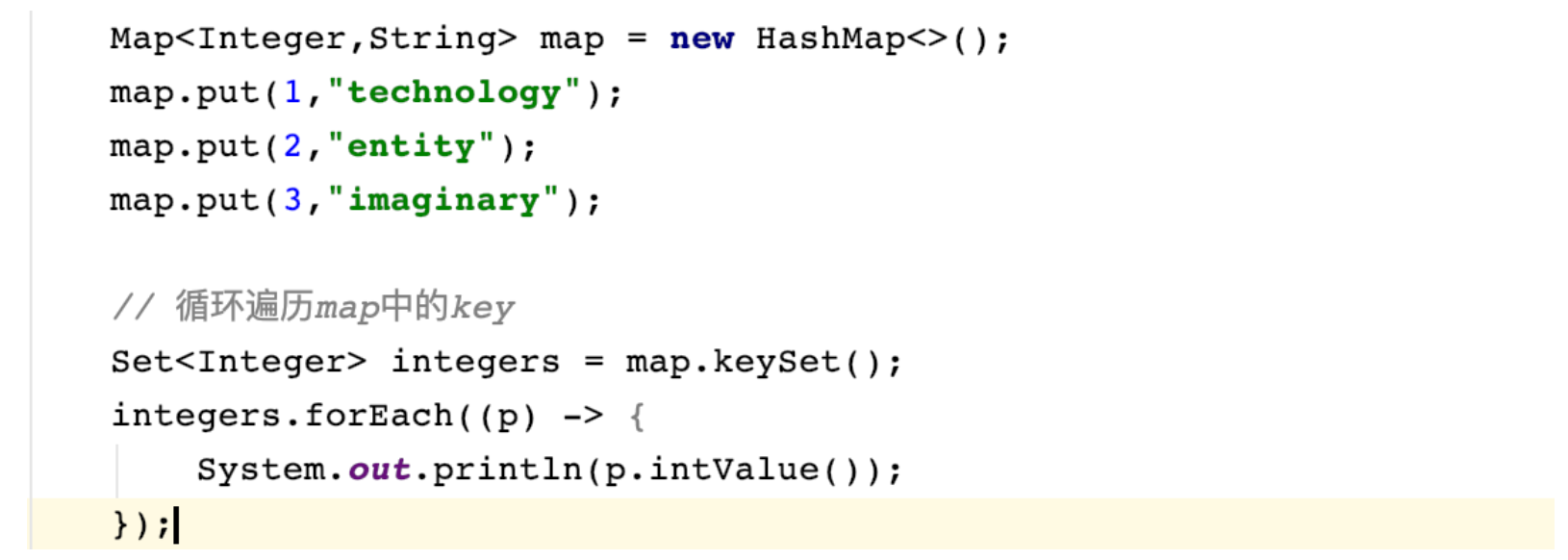// 返回一个set视图，这个视图中包含了map中的key。
public Set<K> keySet() {
// // keySet 指向的是 AbstractMap 中的 keyset
Set<K> ks = keySet;
if (ks == null) {
// 如果 ks 为空，就创建一个 KeySet 对象
// 并对 ks 赋值。
ks = new KeySet();
keySet = ks;
}
return ks;
}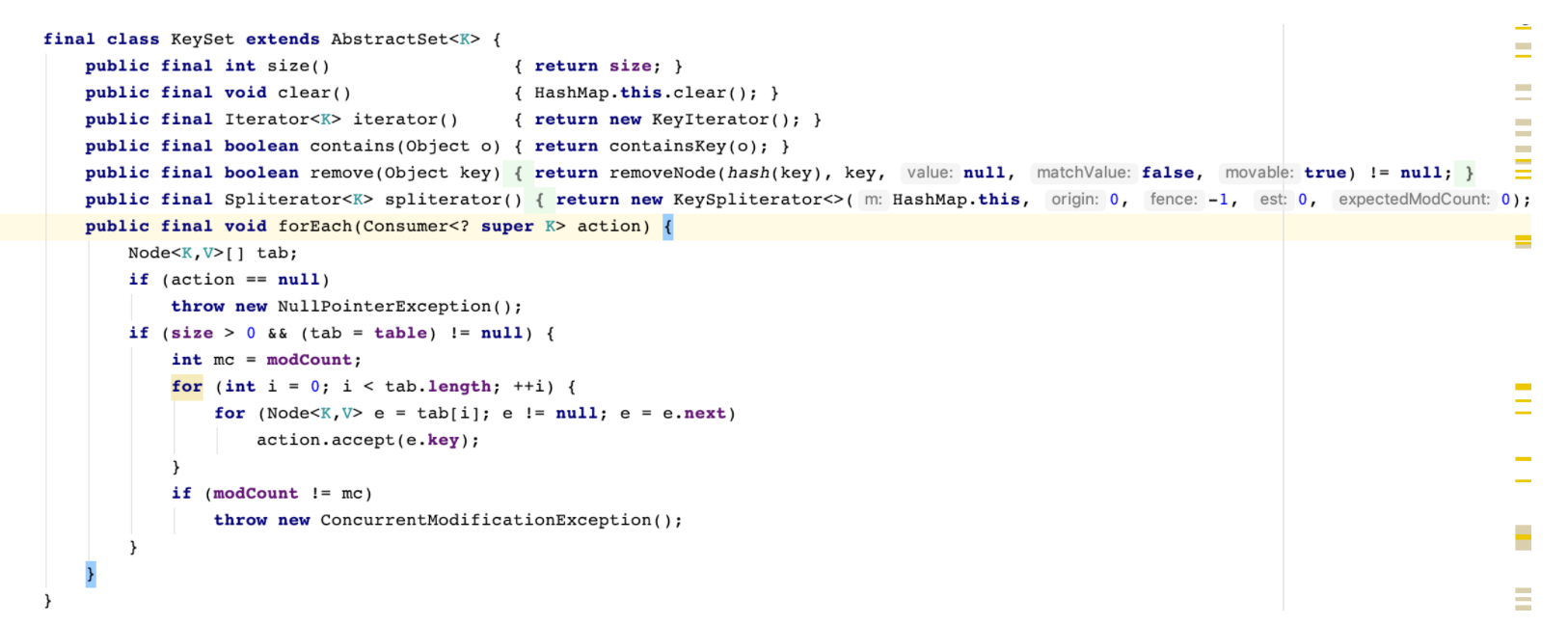#### Values 内部类

Values 类的创建其实是和 KeySet 类很相似，不过 KeySet 旨在对 Map中的键进行操作，Values 旨在对key-value 键值对中的 value 值进行使用，看一下代码示例：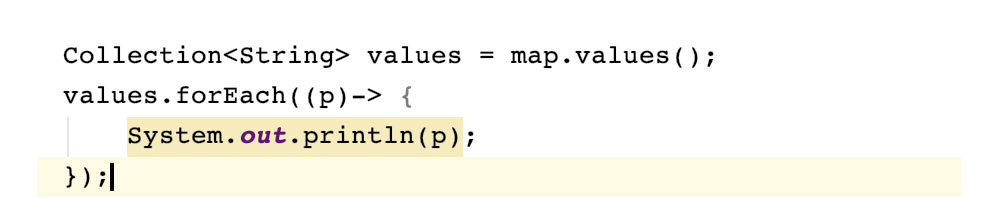public Collection<V> values() {
// values 其实是 AbstractMap 中的 values
Collection<V> vs = values;
if (vs == null) {
vs = new Values();
values = vs;
}
return vs;
}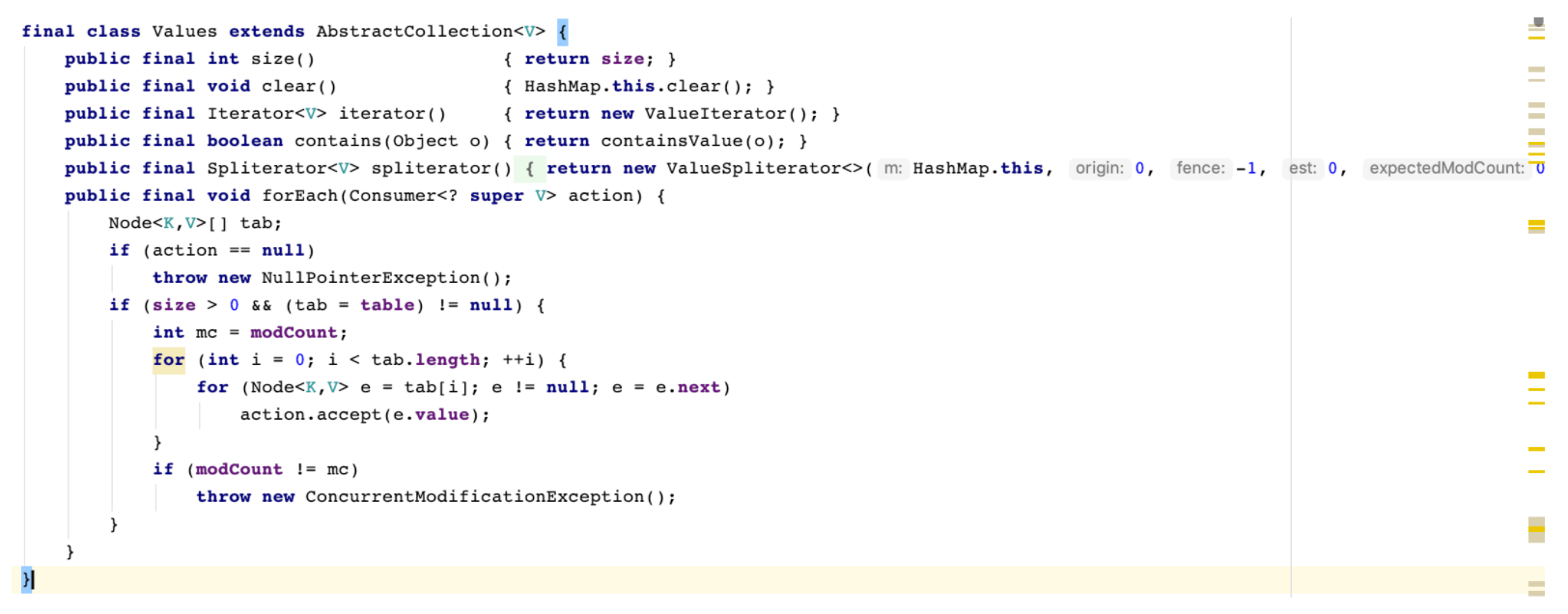#### EntrySet 内部类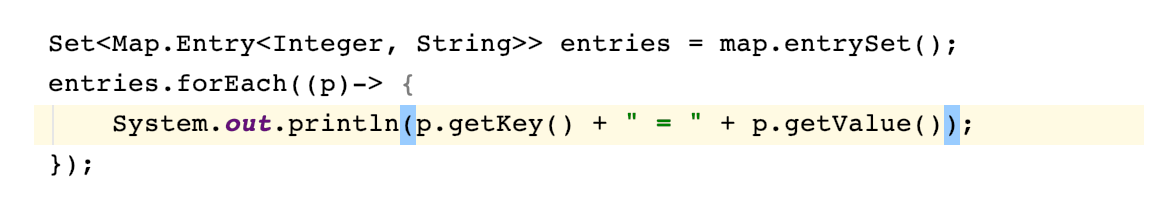// 返回一个 set 视图，此视图包含了 map 中的key-value 键值对
public Set<Map.Entry<K,V>> entrySet() {
Set<Map.Entry<K,V>> es;
return (es = entrySet) == null ? (entrySet = new EntrySet()) : es;
}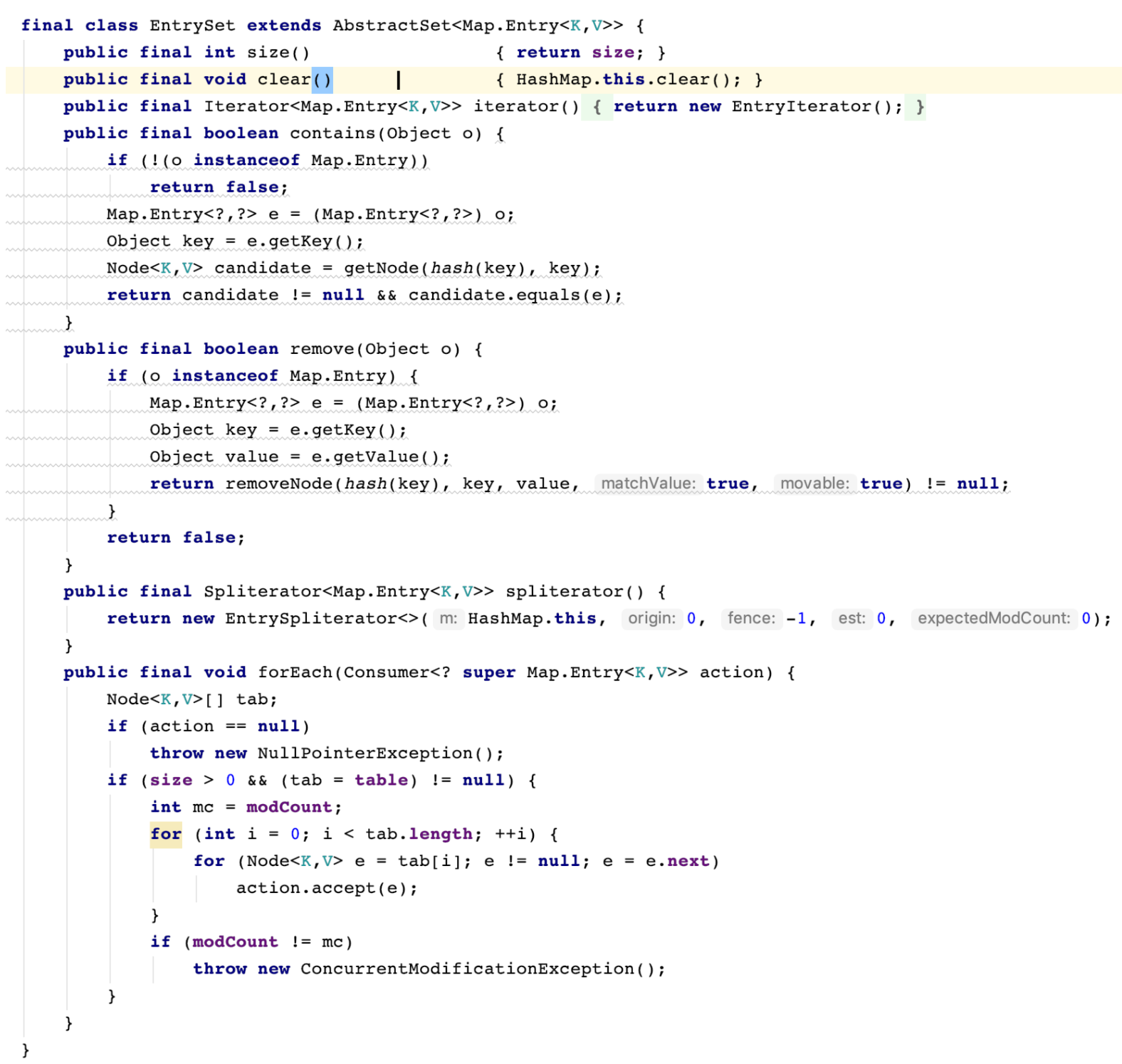### HashMap 1.7 的底层结构

JDK1.7 中，HashMap 采用位桶 + 链表的实现，即使用链表来处理冲突，同一 hash 值的链表都存储在一个数组中。但是当位于一个桶中的元素较多，即 hash 值相等的元素较多时，通过 key 值依次查找的效率较低。它的数据结构如下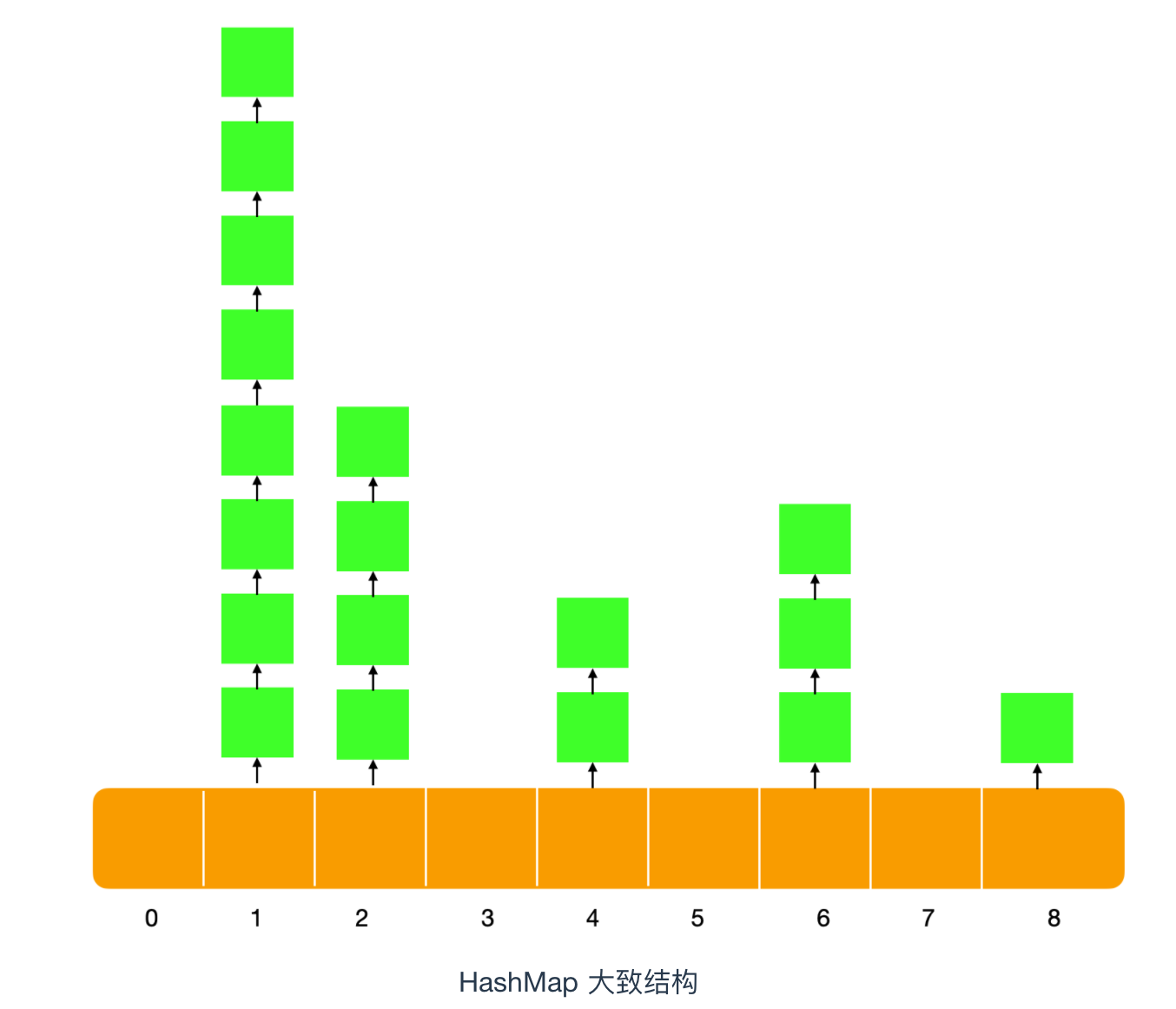HashMap 底层数据结构就是一个 Entry 数组，Entry 是 HashMap 的基本组成单元，每个 Entry 中包含一个 key-value 键值对。

transient Entry<K,V>[] table = (Entry<K,V>[]) EMPTY_TABLE;

static class Entry<K,V> implements Map.Entry<K,V> {
final K key;
V value;
Entry<K,V> next;
int hash;

Entry(int h, K k, V v, Entry<K,V> n) {
value = v;
next = n;
key = k;
hash = h;
}
...
}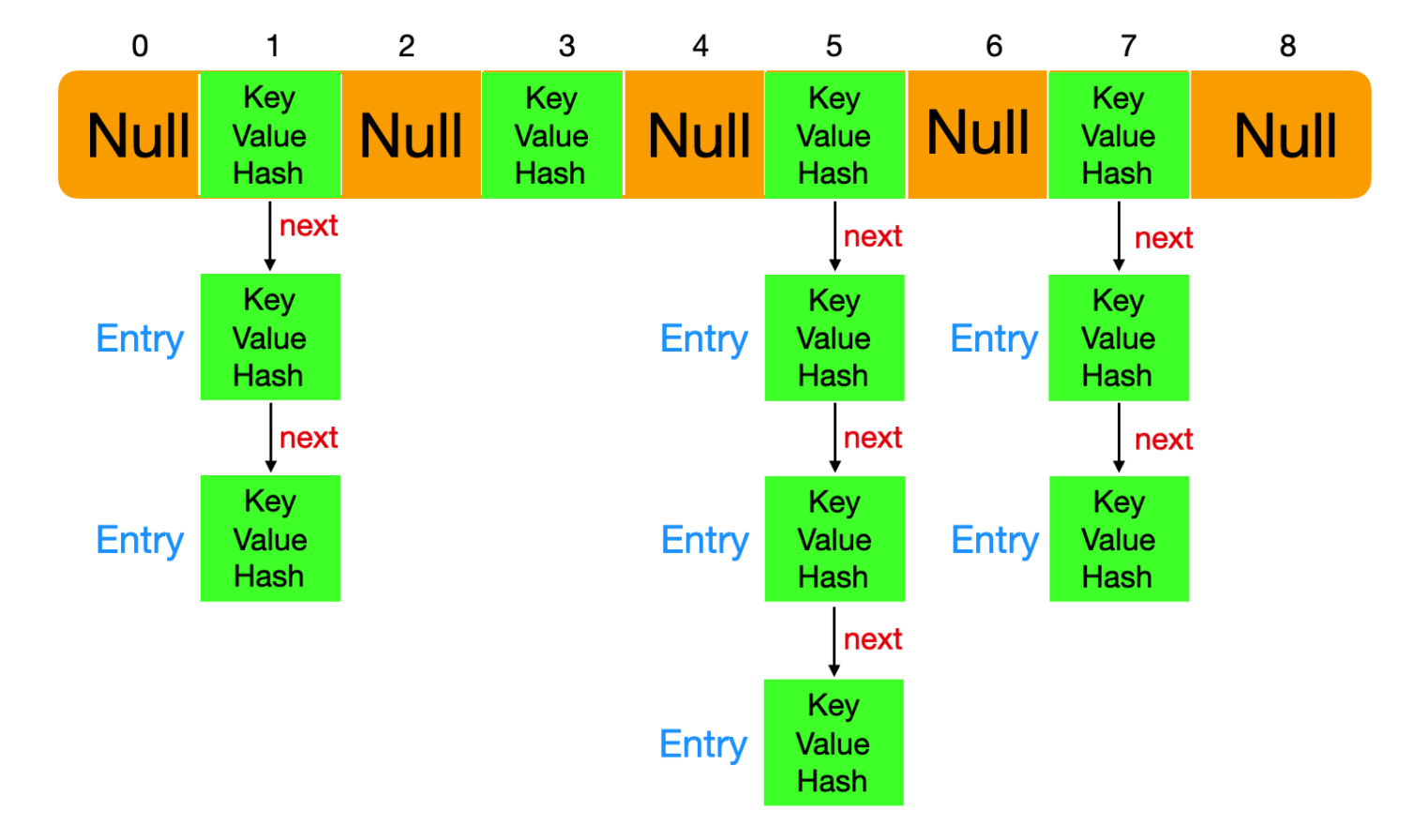### HashMap 1.8 的底层结构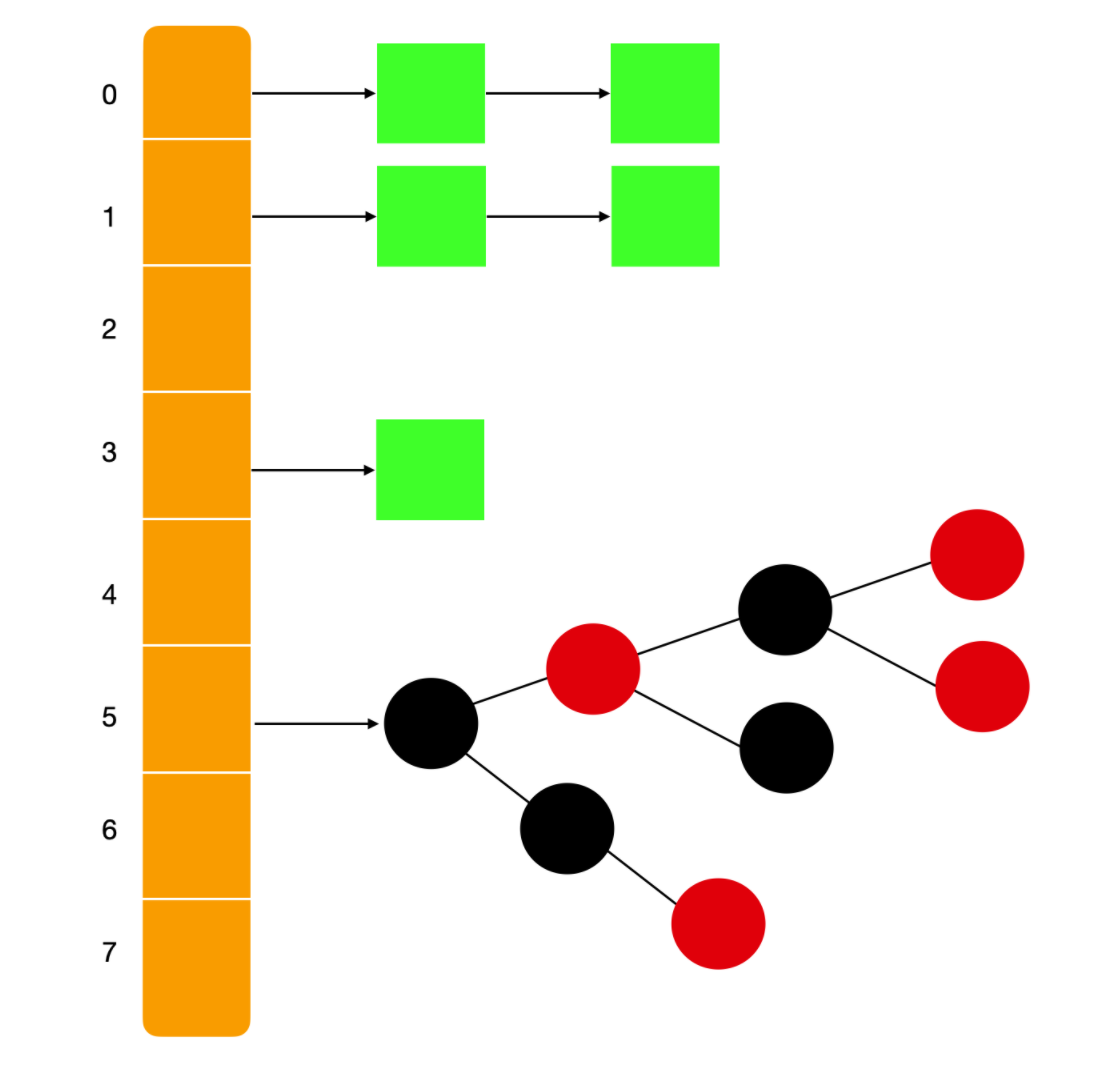### HashMap 重要属性

HashMap 的默认初始容量是由 DEFAULT_INITIAL_CAPACITY 属性管理的。

static final int DEFAULT_INITIAL_CAPACITY = 1 << 4;

HashMaap 的默认初始容量是 1 << 4 = 16， << 是一个左移操作，它相当于是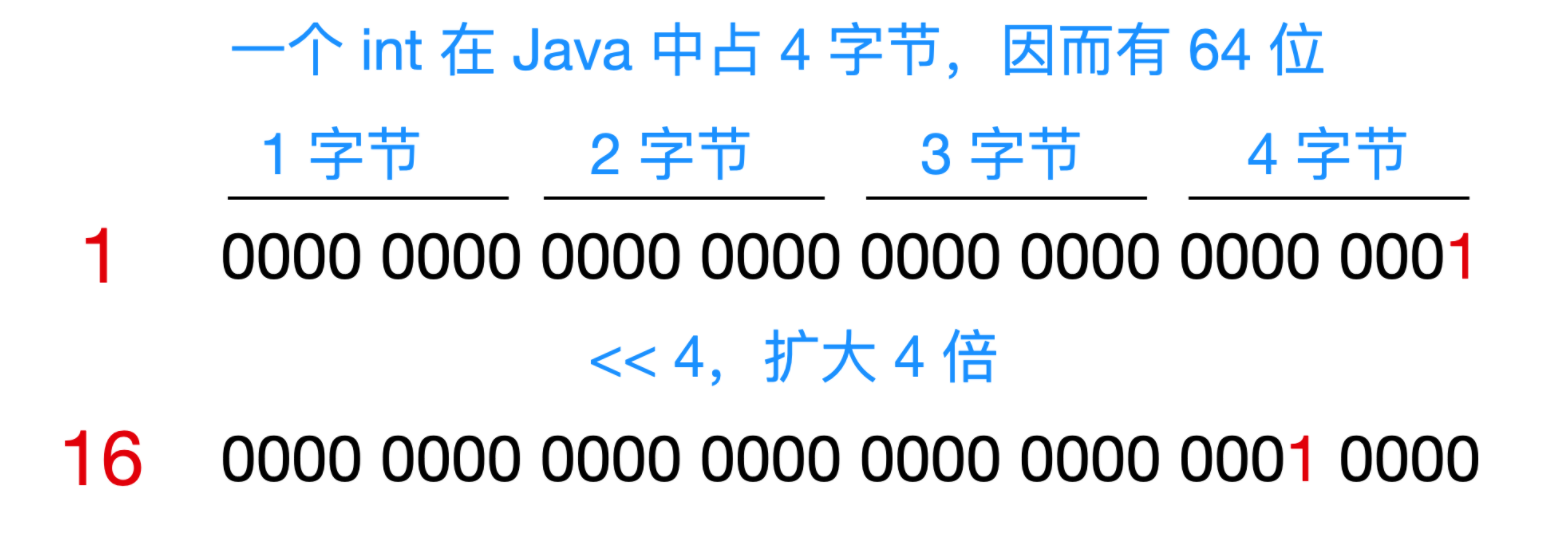HashMap 的最大容量是

static final int MAXIMUM_CAPACITY = 1 << 30;

HashMap 的默认负载因子是

static final float DEFAULT_LOAD_FACTOR = 0.75f;

float 类型所以用 .f 为单位，负载因子是和扩容机制有关，这里大致提一下，后面会细说。扩容机制的原则是当 HashMap 中存储的数量 > HashMap 容量 * 负载因子时，就会把 HashMap 的容量扩大为原来的二倍。

HashMap 的第一次扩容就在 DEFAULT_INITIAL_CAPACITY * DEFAULT_LOAD_FACTOR = 12 时进行。

HashMap 的树化阈值是

static final int TREEIFY_THRESHOLD = 8;

HashMap 的链表阈值是

static final int UNTREEIFY_THRESHOLD = 6;

static final int MIN_TREEIFY_CAPACITY = 64;

HashMap 中的节点数组就是 Entry 数组，它代表的就是 HashMap 中 数组 + 链表 数据结构中的数组。

transient Node<K,V>[] table;

Node 数组在第一次使用的时候进行初始化操作，在必要的时候进行 resize，resize 后数组的长度扩容为原来的二倍。

threshold 涉及到一个扩容的阈值问题，这个问题是由 tableSizeFor 源码解决的。我们先看一下它的源码再来解释

static final int tableSizeFor(int cap) {
int n = cap - 1;
n |= n >>> 1;
n |= n >>> 2;
n |= n >>> 4;
n |= n >>> 8;
n |= n >>> 16;
return (n < 0) ? 1 : (n >= MAXIMUM_CAPACITY) ? MAXIMUM_CAPACITY : n + 1;
}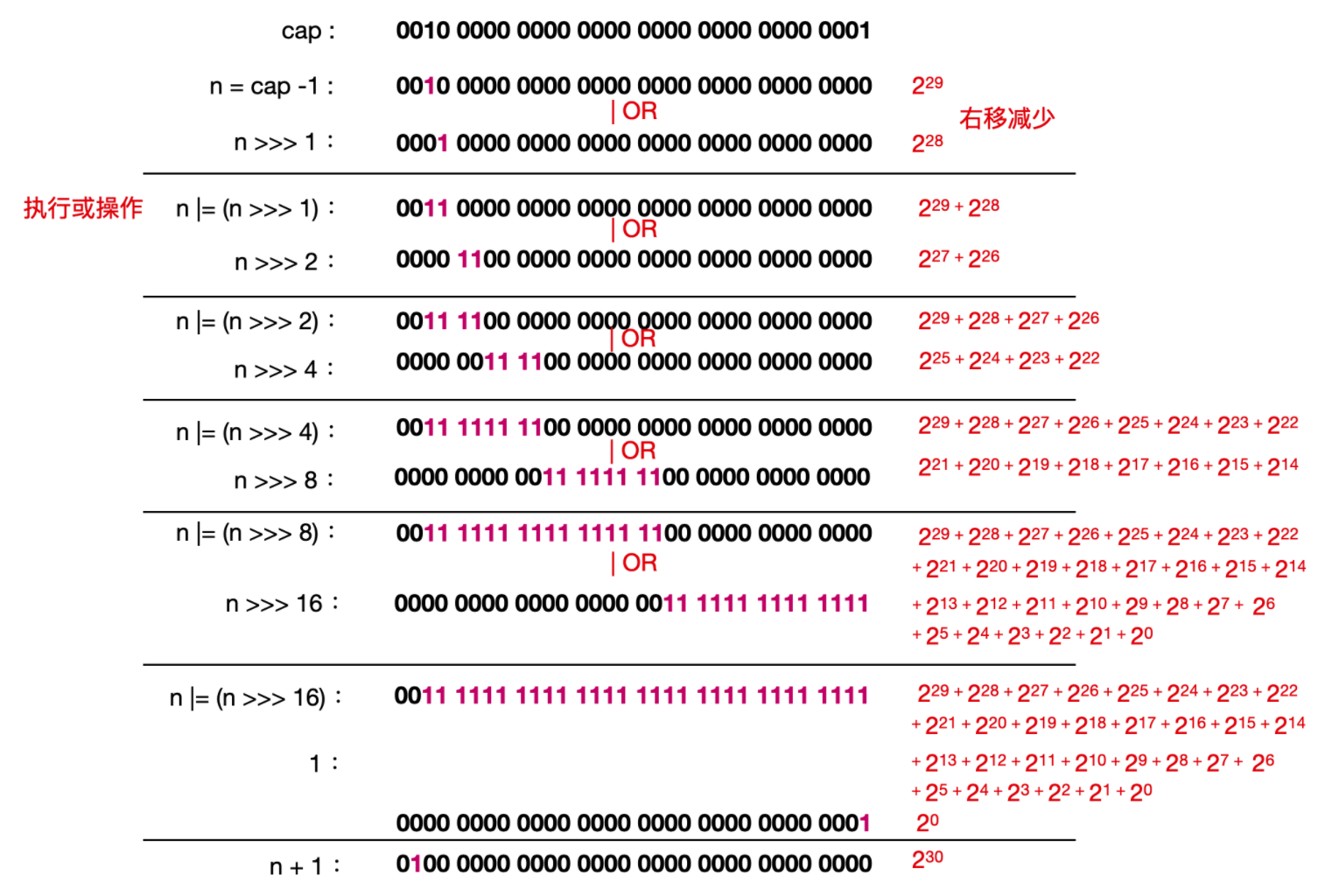loadFactor 表示负载因子，它表示的是 HashMap 中的密集程度。

### HashMap 构造函数

• 带有初始容量 initialCapacity负载因子 loadFactor 的构造函数
public HashMap(int initialCapacity, float loadFactor) {
if (initialCapacity < 0)
throw new IllegalArgumentException("Illegal initial capacity: " +
initialCapacity);
if (initialCapacity > MAXIMUM_CAPACITY)
initialCapacity = MAXIMUM_CAPACITY;
throw new IllegalArgumentException("Illegal load factor: " +
// 扩容的阈值
this.threshold = tableSizeFor(initialCapacity);
}

• 只带有 initialCapacity 的构造函数
public HashMap(int initialCapacity) {
}

• 无参数的构造函数
public HashMap() {
}

• 带有 map 的构造函数
public HashMap(Map<? extends K, ? extends V> m) {
putMapEntries(m, false);
}

### 讲一讲 HashMap put 的全过程

final V putVal(int hash, K key, V value, boolean onlyIfAbsent,
boolean evict) {
Node<K,V>[] tab; Node<K,V> p; int n, i;
// 如果table 为null 或者没有为 table 分配内存，就resize一次
if ((tab = table) == null || (n = tab.length) == 0)
n = (tab = resize()).length;
// 指定hash值节点为空则直接插入，这个(n - 1) & hash才是表中真正的哈希
if ((p = tab[i = (n - 1) & hash]) == null)
tab[i] = newNode(hash, key, value, null);
// 如果不为空
else {
Node<K,V> e; K k;
// 计算表中的这个真正的哈希值与要插入的key.hash相比
if (p.hash == hash &&
((k = p.key) == key || (key != null && key.equals(k))))
e = p;
// 若不同的话，并且当前节点已经在 TreeNode 上了
else if (p instanceof TreeNode)
// 采用红黑树存储方式
e = ((TreeNode<K,V>)p).putTreeVal(this, tab, hash, key, value);
// key.hash 不同并且也不再 TreeNode 上，在链表上找到 p.next==null
else {
for (int binCount = 0; ; ++binCount) {
if ((e = p.next) == null) {
// 在表尾插入
p.next = newNode(hash, key, value, null);
// 新增节点后如果节点个数到达阈值，则进入 treeifyBin() 进行再次判断
if (binCount >= TREEIFY_THRESHOLD - 1) // -1 for 1st
treeifyBin(tab, hash);
break;
}
// 如果找到了同 hash、key 的节点，那么直接退出循环
if (e.hash == hash &&
((k = e.key) == key || (key != null && key.equals(k))))
break;
// 更新 p 指向下一节点
p = e;
}
}
// map中含有旧值，返回旧值
if (e != null) { // existing mapping for key
V oldValue = e.value;
if (!onlyIfAbsent || oldValue == null)
e.value = value;
afterNodeAccess(e);
return oldValue;
}
}
// map调整次数 + 1
++modCount;
// 键值对的数量达到阈值，需要扩容
if (++size > threshold)
resize();
afterNodeInsertion(evict);
return null;
}

• hash -> put 放在桶中的位置，在 put 之前，会进行 hash 函数的计算。
• key -> 参数的 key 值
• value -> 参数的 value 值
• onlyIfAbsent -> 是否改变已经存在的值，也就是是否进行 value 值的替换标志
• evict -> 是否是刚创建 HashMap 的标志

public V put(K key, V value) {
return putVal(hash(key), key, value, false, true);
}

static final int hash(Object key) {
int h;
return (key == null) ? 0 : (h = key.hashCode()) ^ (h >>> 16);
}

#### Hash 函数

hash 函数会根据你传递的 key 值进行计算，首先计算 key 的 hashCode 值，然后再对 hashcode 进行无符号右移操作，最后再和 hashCode 进行异或 ^ 操作。

>>>: 无符号右移操作，它指的是 无符号右移，也叫逻辑右移，即若该数为正，则高位补0，而若该数为负数，则右移后高位同样补0 ，也就是不管是正数还是负数，右移都会在空缺位补 0 。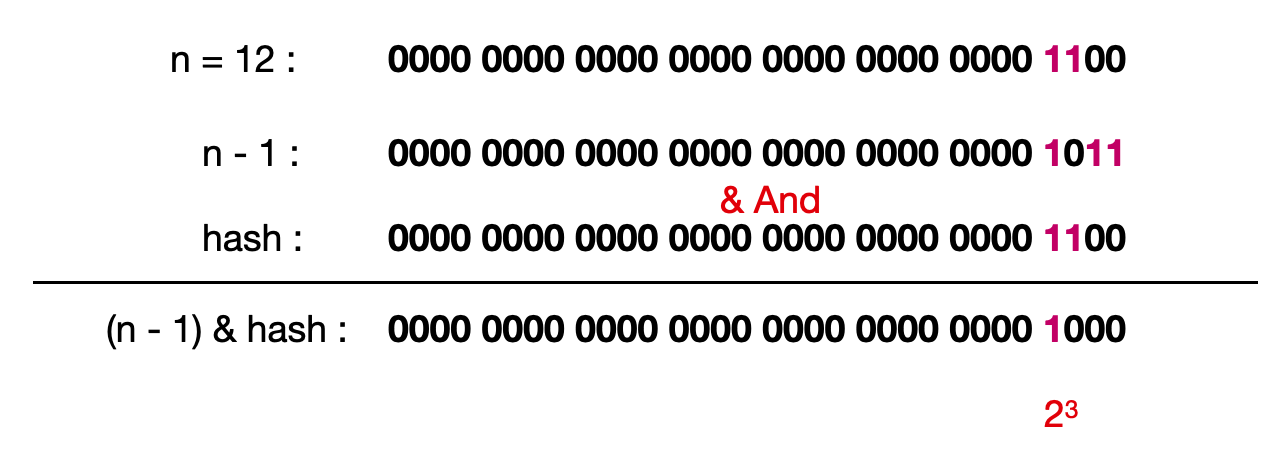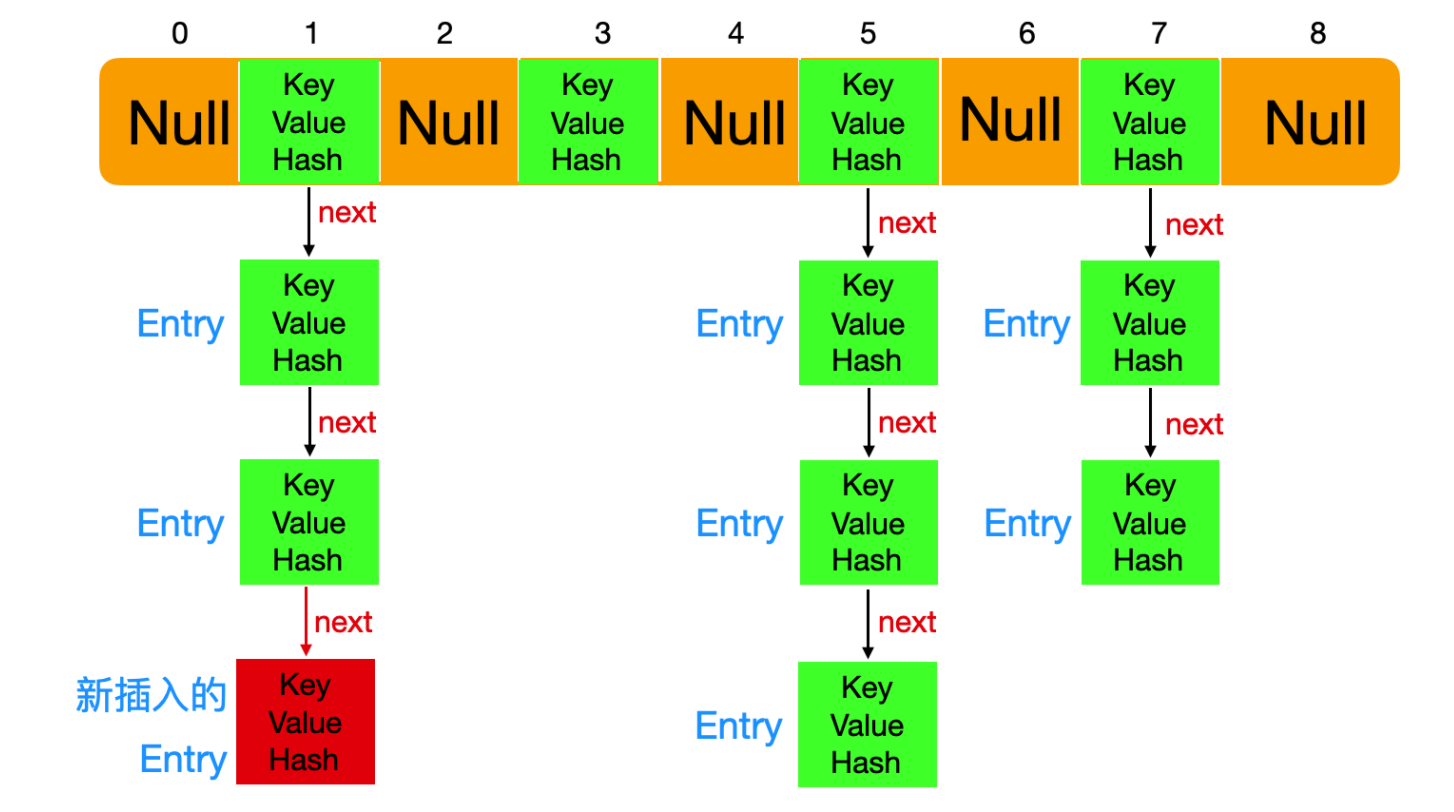### 扩容机制

final Node<K,V>[] resize() {
Node<K,V>[] oldTab = table;
// 存储old table 的大小
int oldCap = (oldTab == null) ? 0 : oldTab.length;
// 存储扩容阈值
int oldThr = threshold;
int newCap, newThr = 0;
if (oldCap > 0) {
// 如果old table数据已达最大，那么threshold也被设置成最大
if (oldCap >= MAXIMUM_CAPACITY) {
threshold = Integer.MAX_VALUE;
return oldTab;
}
// 左移扩大二倍,
else if ((newCap = oldCap << 1) < MAXIMUM_CAPACITY &&
oldCap >= DEFAULT_INITIAL_CAPACITY)
// 扩容成原来二倍
newThr = oldThr << 1; // double threshold
}
// 如果oldThr                                                                                                                                               !> 0
else if (oldThr > 0) // initial capacity was placed in threshold
newCap = oldThr;
// 如果old table <= 0 并且 存储的阈值 <= 0
else {               // zero initial threshold signifies using defaults
newCap = DEFAULT_INITIAL_CAPACITY;
}
// 如果扩充阈值为0
if (newThr == 0) {
// 扩容阈值为 初始容量*负载因子
float ft = (float)newCap * loadFactor;
newThr = (newCap < MAXIMUM_CAPACITY && ft < (float)MAXIMUM_CAPACITY ?
(int)ft : Integer.MAX_VALUE);
}
// 重新给负载因子赋值
threshold = newThr;
// 获取扩容后的数组
@SuppressWarnings({"rawtypes","unchecked"})
Node<K,V>[] newTab = (Node<K,V>[])new Node[newCap];
table = newTab;
// 如果第一次进行table 初始化不会走下面的代码
// 扩容之后需要重新把节点放在新扩容的数组中
if (oldTab != null) {
for (int j = 0; j < oldCap; ++j) {
Node<K,V> e;
if ((e = oldTab[j]) != null) {
oldTab[j] = null;
if (e.next == null)
newTab[e.hash & (newCap - 1)] = e;
else if (e instanceof TreeNode)
// 重新映射时，需要对红黑树进行拆分
((TreeNode<K,V>)e).split(this, newTab, j, oldCap);
else { // preserve order
Node<K,V> loHead = null, loTail = null;
Node<K,V> hiHead = null, hiTail = null;
Node<K,V> next;
// 遍历链表，并将链表节点按原顺序进行分组
do {
next = e.next;
if ((e.hash & oldCap) == 0) {
if (loTail == null)
else
loTail.next = e;
loTail = e;
}
else {
if (hiTail == null)
else
hiTail.next = e;
hiTail = e;
}
} while ((e = next) != null);
// 将分组后的链表映射到新桶中
if (loTail != null) {
loTail.next = null;
}
if (hiTail != null) {
hiTail.next = null;
}
}
}
}
}
return newTab;
}

• 判断 HashMap 中的数组的长度，也就是 (Node<K,V>[])oldTab.length() ，再判断数组的长度是否比最大的的长度也就是 2^30 次幂要大，大的话直接取最大长度，否则利用位运算 <<扩容为原来的两倍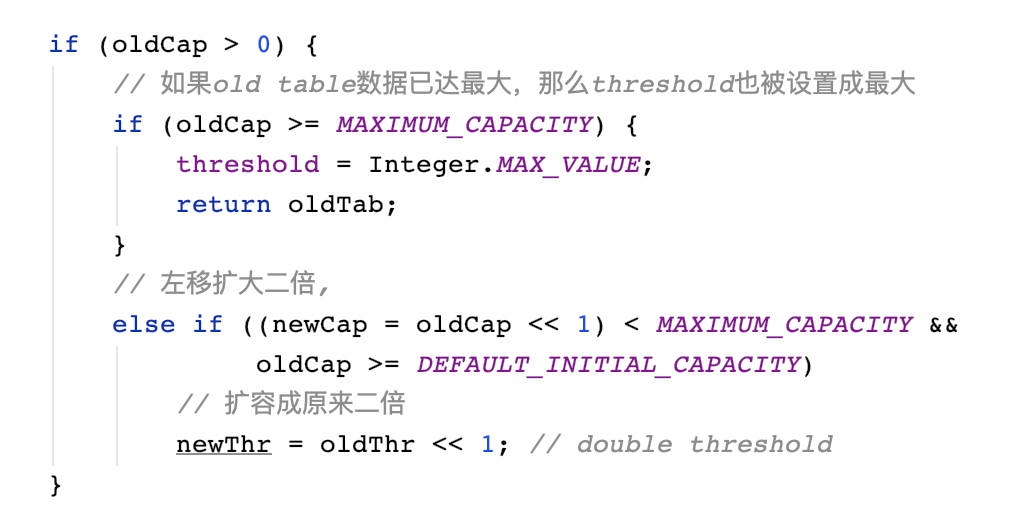• 如果数组长度不大于0 ，再判断扩容阈值 threshold 是否大于 0 ，也就是看有无外部指定的扩容阈值，若有则使用，这里需要说明一下 threshold 何时是 oldThr > 0 ，因为 oldThr = threshold ，这里其实比较的就是 threshold，因为 HashMap 中的每个构造方法都会调用 HashMap(initCapacity,loadFactor) 这个构造方法，所以如果没有外部指定 initialCapacity，初始容量使用的就是 16，然后根据 this.threshold = tableSizeFor(initialCapacity); 求得 threshold 的值。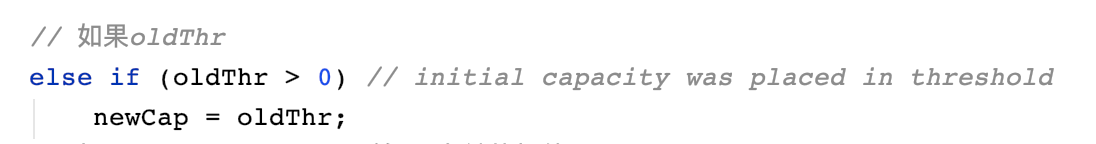• 否则，直接使用默认的初始容量和扩容阈值，走 else 的逻辑是在 table 刚刚初始化的时候。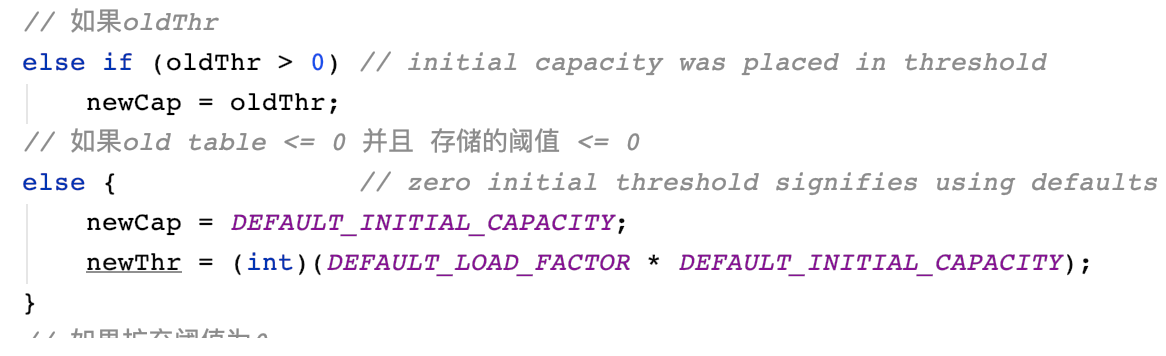• 循环桶中的每个 Node 节点，判断 Node[i] 是否为空，为空直接返回，不为空则遍历桶数组，并将键值对映射到新的桶数组中。

• 如果不为空，再判断是否是树形结构，如果是树形结构则按照树形结构进行拆分，拆分方法在 split 方法中。

• 如果不是树形结构，则遍历链表，并将链表节点按原顺序进行分组。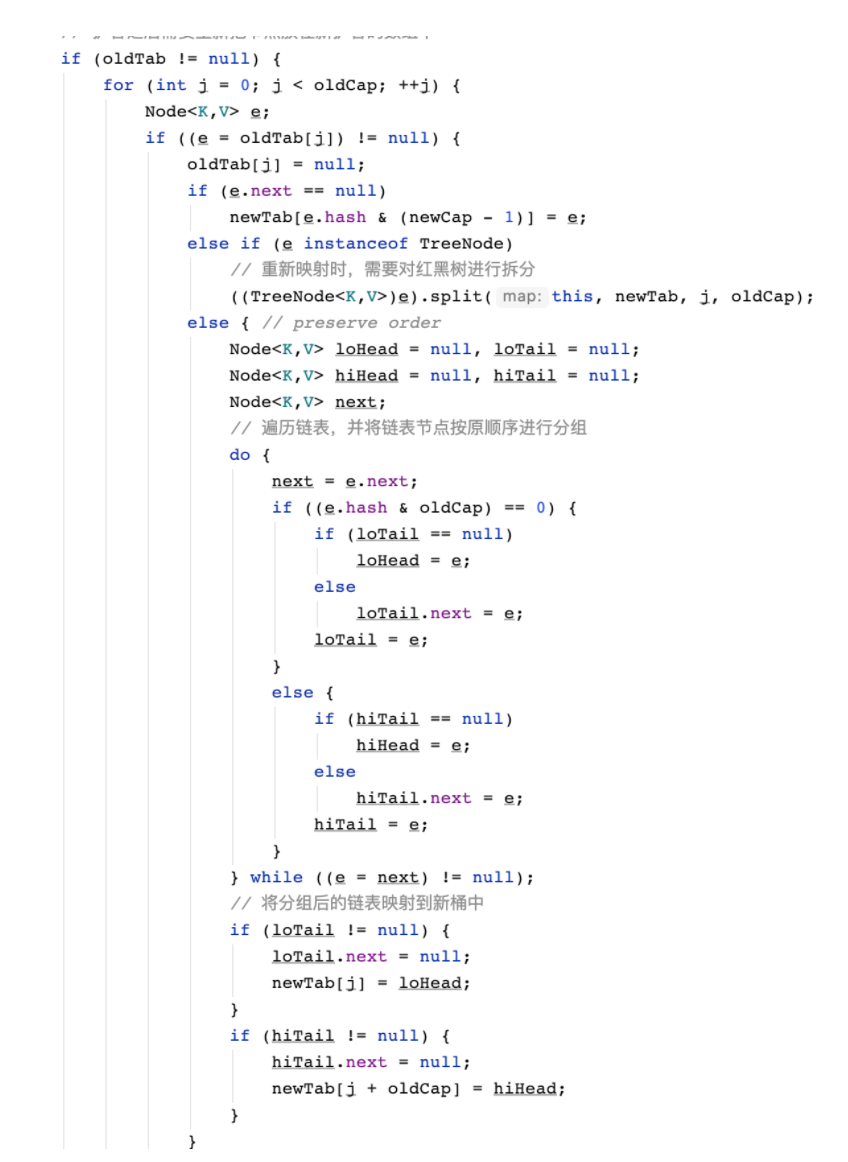### 讲一讲 get 方法全过程

public V get(Object key) {
Node<K,V> e;
return (e = getNode(hash(key), key)) == null ? null : e.value;
}

final Node<K,V> getNode(int hash, Object key) {
Node<K,V>[] tab; Node<K,V> first, e; int n; K k;

// 找到真实的元素位置
if ((tab = table) != null && (n = tab.length) > 0 &&
(first = tab[(n - 1) & hash]) != null) {
// 总是会check 一下第一个元素
if (first.hash == hash && // always check first node
((k = first.key) == key || (key != null && key.equals(k))))
return first;

// 如果不是第一个元素，并且下一个元素不是空的
if ((e = first.next) != null) {

// 判断是否属于 TreeNode，如果是 TreeNode 实例，直接从 TreeNode.getTreeNode 取
if (first instanceof TreeNode)
return ((TreeNode<K,V>)first).getTreeNode(hash, key);

// 如果还不是 TreeNode 实例，就直接循环数组元素，直到找到指定元素位置
do {
if (e.hash == hash &&
((k = e.key) == key || (key != null && key.equals(k))))
return e;
} while ((e = e.next) != null);
}
}
return null;
}


getNode 方法有一个比较重要的过程就是 (n – 1) & hash，这段代码是确定需要查找的桶的位置的，那么，为什么要 (n – 1) & hash 呢？

n 就是 HashMap 中桶的数量，这句话的意思也就是说 (n – 1) & hash 就是 (桶的容量 – 1) & hash

// 为什么 HashMap 的检索位置是 (table.size - 1) & hash
public static void main(String[] args) {

Map<String,Object> map = new HashMap<>();

// debug 得知 1 的 hash 值算出来是 49
map.put("1","cxuan");
// debug 得知 1 的 hash 值算出来是 50
map.put("2","cxuan");
// debug 得知 1 的 hash 值算出来是 51
map.put("3","cxuan");

}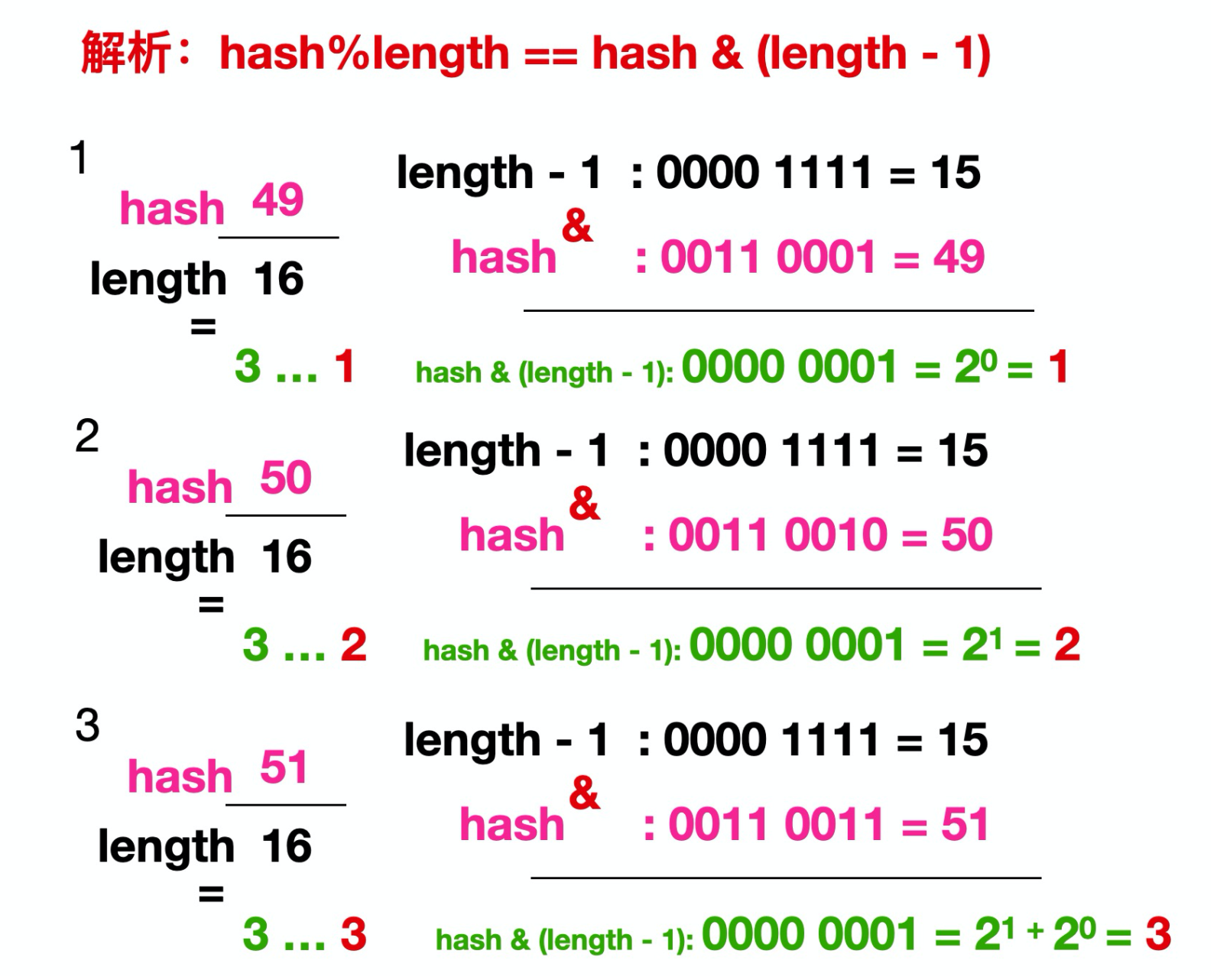### HashMap 的遍历方式

HashMap 的遍历，也是一个使用频次特别高的操作

HashMap 遍历的基类是 HashIterator，它是一个 Hash 迭代器，它是一个 HashMap 内部的抽象类，它的构造比较简单，只有三种方法，hasNext 、 remove 和 nextNode 方法，其中 nextNode 方法是由三种迭代器实现的

• KeyIterator ，对 key 进行遍历
• ValueIterator，对 value 进行遍历
• EntryIterator， 对 Entry 链进行遍历

final class KeyIterator extends HashIterator
implements Iterator<K> {
public final K next() { return nextNode().key; }
}

final class ValueIterator extends HashIterator
implements Iterator<V> {
public final V next() { return nextNode().value; }
}

final class EntryIterator extends HashIterator
implements Iterator<Map.Entry<K,V>> {
public final Map.Entry<K,V> next() { return nextNode(); }
}

HashIterator 中的遍历方式

abstract class HashIterator {
Node<K,V> next;        // 下一个 entry 节点
Node<K,V> current;     // 当前 entry 节点
int expectedModCount;  // fail-fast 的判断标识
int index;             // 当前槽

HashIterator() {
expectedModCount = modCount;
Node<K,V>[] t = table;
current = next = null;
index = 0;
if (t != null && size > 0) { // advance to first entry
do {} while (index < t.length && (next = t[index++]) == null);
}
}

public final boolean hasNext() {
return next != null;
}

final Node<K,V> nextNode() {
Node<K,V>[] t;
Node<K,V> e = next;
if (modCount != expectedModCount)
throw new ConcurrentModificationException();
if (e == null)
throw new NoSuchElementException();
if ((next = (current = e).next) == null && (t = table) != null) {
do {} while (index < t.length && (next = t[index++]) == null);
}
return e;
}

public final void remove() {...}
}

next 和 current 分别表示下一个 Node 节点和当前的 Node 节点，HashIterator 在初始化时会遍历所有的节点。下面我们用图来表示一下他们的遍历顺序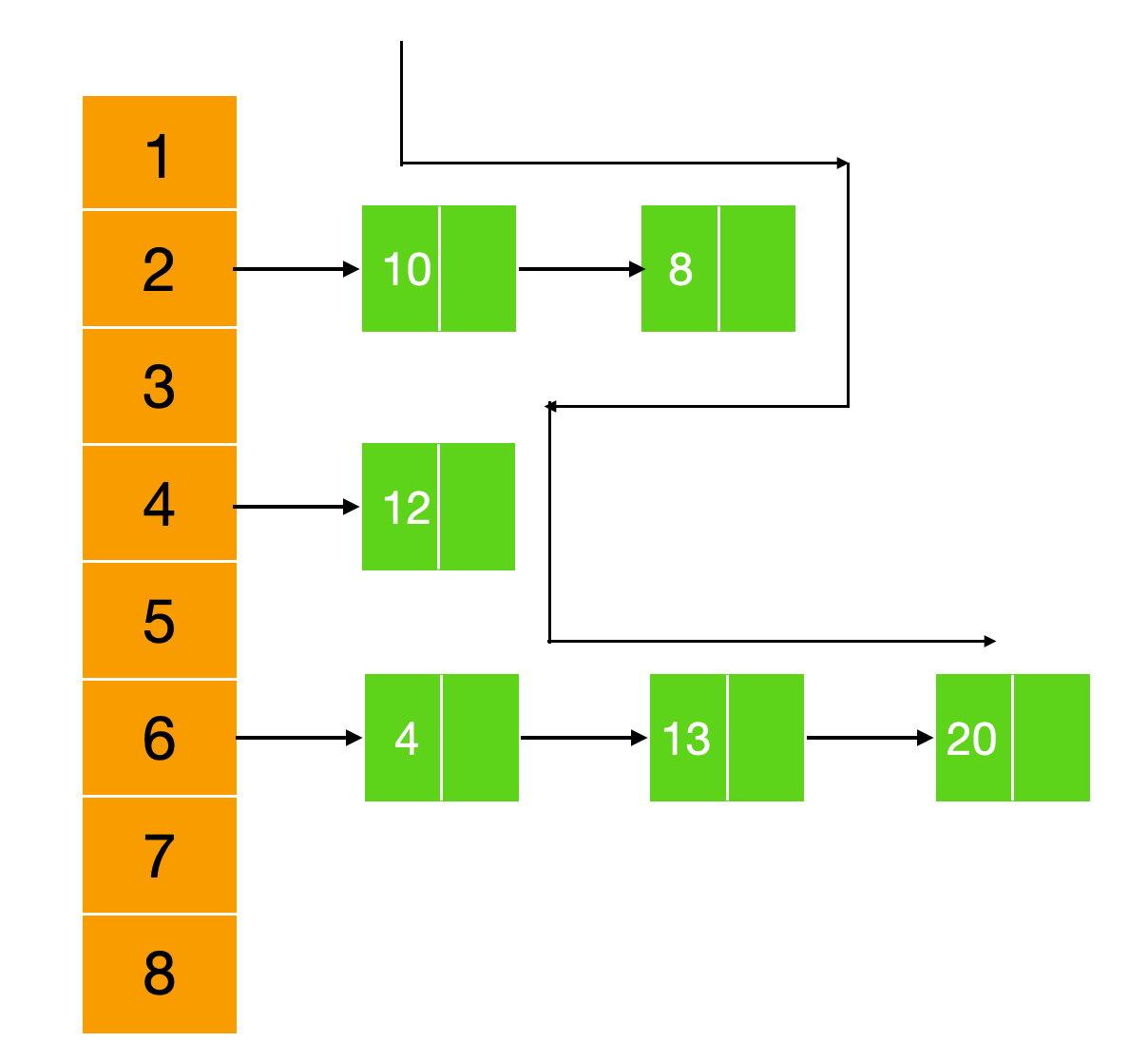### HashMap 中的移除方法

HashMap 中的移除方法也比较简单了，源码如下

public V remove(Object key) {
Node<K,V> e;
return (e = removeNode(hash(key), key, null, false, true)) == null ?
null : e.value;
}

final Node<K,V> removeNode(int hash, Object key, Object value,
boolean matchValue, boolean movable) {
Node<K,V>[] tab; Node<K,V> p; int n, index;
if ((tab = table) != null && (n = tab.length) > 0 &&
(p = tab[index = (n - 1) & hash]) != null) {
Node<K,V> node = null, e; K k; V v;
if (p.hash == hash &&
((k = p.key) == key || (key != null && key.equals(k))))
node = p;
else if ((e = p.next) != null) {
if (p instanceof TreeNode)
node = ((TreeNode<K,V>)p).getTreeNode(hash, key);
else {
do {
if (e.hash == hash &&
((k = e.key) == key ||
(key != null && key.equals(k)))) {
node = e;
break;
}
p = e;
} while ((e = e.next) != null);
}
}
if (node != null && (!matchValue || (v = node.value) == value ||
(value != null && value.equals(v)))) {
if (node instanceof TreeNode)
((TreeNode<K,V>)node).removeTreeNode(this, tab, movable);
else if (node == p)
tab[index] = node.next;
else
p.next = node.next;
++modCount;
--size;
afterNodeRemoval(node);
return node;
}
}
return null;
}

remove 方法有很多，最终都会调用到 removeNode 方法，只不过传递的参数值不同，我们拿 remove(object) 来演示一下。

## 关于 HashMap 的面试题

### HashMap 的数据结构

JDK1.7 中，HashMap 采用位桶 + 链表的实现，即使用链表来处理冲突，同一 hash 值的链表都存储在一个数组中。但是当位于一个桶中的元素较多，即 hash 值相等的元素较多时，通过 key 值依次查找的效率较低。

### HashMap 为啥线程不安全

HashMap 不是一个线程安全的容器，不安全性体现在多线程并发对 HashMap 进行 put 操作上。如果有两个线程 A 和 B ，首先 A 希望插入一个键值对到 HashMap 中，在决定好桶的位置进行 put 时，此时 A 的时间片正好用完了，轮到 B 运行，B 运行后执行和 A 一样的操作，只不过 B 成功把键值对插入进去了。如果 A 和 B 插入的位置（桶）是一样的，那么线程 A 继续执行后就会覆盖 B 的记录，造成了数据不一致问题。

### HashMap 是如何处理哈希碰撞的

HashMap 底层是使用位桶 + 链表实现的，位桶决定元素的插入位置，位桶是由 hash 方法决定的，当多个元素的 hash 计算得到相同的哈希值后，HashMap 会把多个 Node 元素都放在对应的位桶中，形成链表，这种处理哈希碰撞的方式被称为链地址法。

### HashMap 是如何扩容的

HashMap 中有两个非常重要的变量，一个是 loadFactor ，一个是 threshold ，loadFactor 表示的就是负载因子，threshold 表示的是下一次要扩容的阈值，当 threshold = loadFactor * 数组长度时，数组长度扩大位原来的两倍，来重新调整 map 的大小，并将原来的对象放入新的 bucket 数组中。

### HashMap 线程安全的实现有哪些

Collections.synchronizedMap(new HashMap());